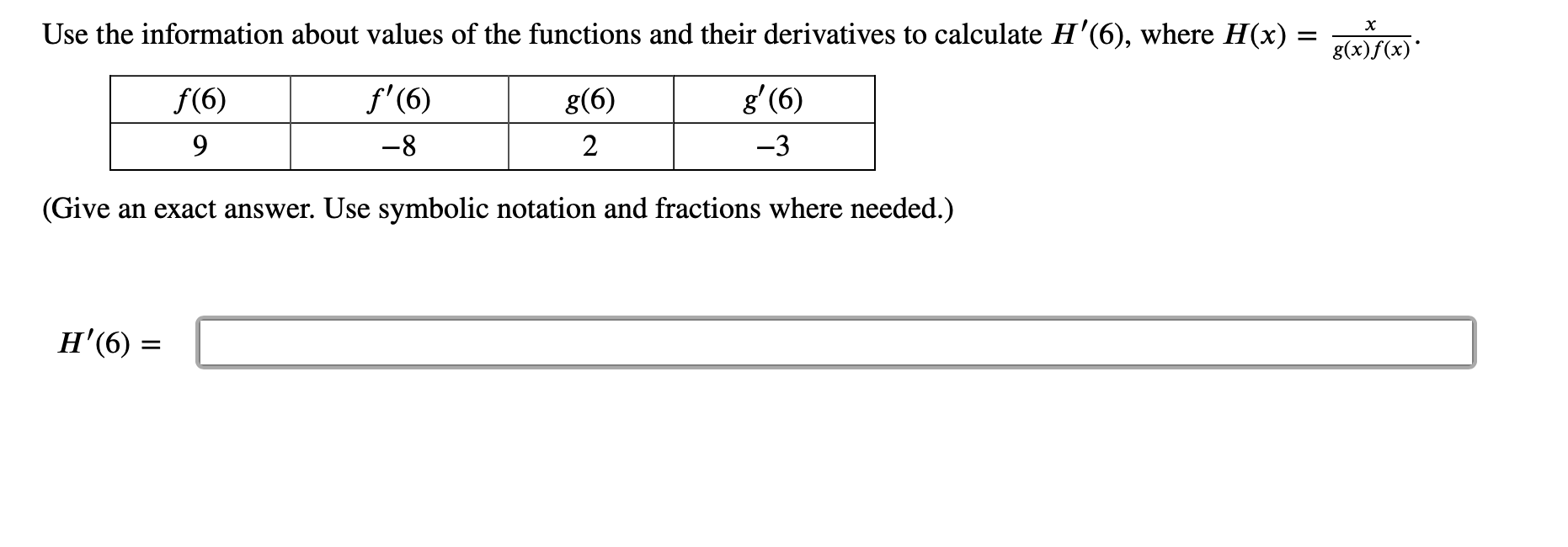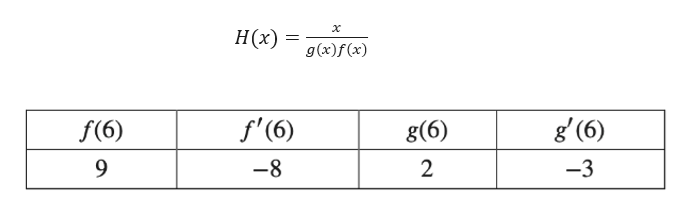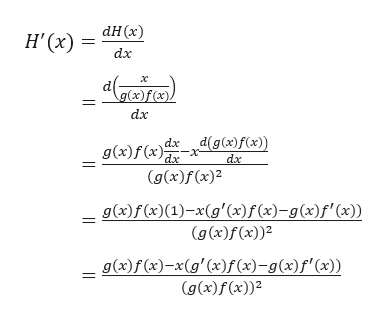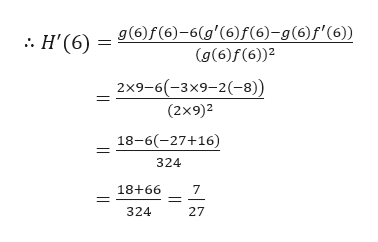Use the information about values of the functions and their derivatives to calculate H'(6), where H(x)хf'(6)g' (6)f(6)g(6)9-82-3(Give an exact answer. Use symbolic notation and fractions where needed.)Н (6)

Questionhelp_outlineImage TranscriptioncloseUse the information about values of the functions and their derivatives to calculate H'(6), where H(x) х f'(6) g' (6) f(6) g(6) 9 -8 2 -3 (Give an exact answer. Use symbolic notation and fractions where needed.) Н (6) fullscreen
Step 1

Given,help_outlineImage Transcriptioncloseх Н(2) g(x)f(x) g (6) f'(6) f(6) g(6) 9 -8 2 -3 fullscreen
Step 2

Now, differentiating the H(x) with respect to x, we gethelp_outlineImage TranscriptioncloseаH (x) Н'(x) dx х g(x)f (x). dx g(x)f(x)ddg(x)f(x2)) dx -x- (g(x)f (x)2 g(x)f(x)(1)-x(g'(x)f (x)-g(x)f'(x)) (g (x)f(x))2 g(x)f (x)-x(g' (x)f(x)-g(x)f'(x)) (g(x)f(x))2 fullscreen
Step 3

Now, putting the required values from the ...help_outlineImage Transcriptioncloseg(6)f(6)-6(g'(6)f(6)-g(6)f'(6)) (g(6)f(6)2 :. H'(6) = 2x9-6(-3x9-2(-8)) (2x9)2 18-6(-27+16) 324 18+66 324 27 fullscreen

Want to see the full answer?

See Solution

Want to see this answer and more?

Our solutions are written by experts, many with advanced degrees, and available 24/7

See Solution
Tagged in

Derivative